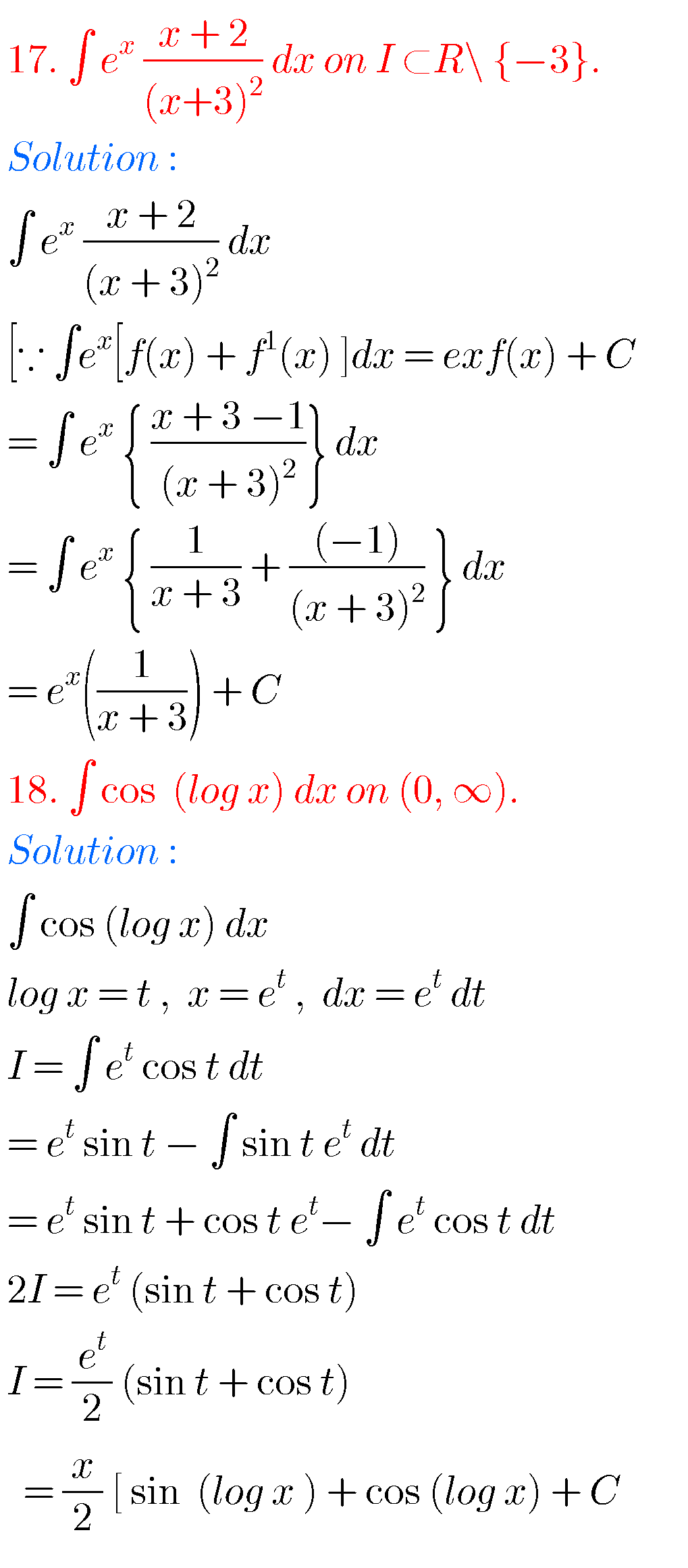## Intermediate Maths solutions for Integration

Intermediate Maths solutions for Integration Inter mathematics 2b solutions for Integration exercise 6(a), 6(b), 6(c), 6(d), 6(e) and 6(f) are given. These solutions are very easy to understand. First you study the textbook lesson very well. Observe the example problems and solutions given in the textbook. Try them. Observe the solutions given below and try …